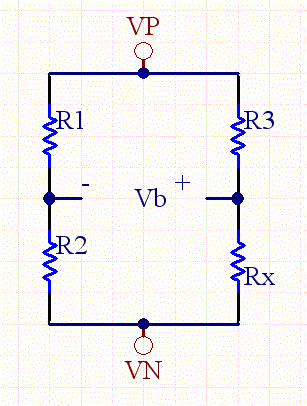Daycounter, Inc. Engineering Services Custom Firmware, Electronics Design, and PCB Layout Home Company Services Products Partners Clients Site Map Contact UsWheatstone Bridge CalculatorSee our other Electronics Calculators.

The Wheatstone bridge is a very useful circuit.  When the bridge is fully balanced, the right side resistors identical to the left side resistors (R1=R3, and R2=Rx), the voltage across the bridge is zero.  However, with a small change in resistance on one of the resistors, and the bridge becomes unbalanced, and a voltage difference appears. Wheatstone bridge are used in all sorts of sensor devices such as strain gauges, pressure gauges, etc.A differencing amplifier can be used to extract the common mode signal, while rejecting all of the common mode noise.  As a result very small signals and changes can be extracted from the bridge, because common mode noise is easily rejected.

 Inputs: Vin: Input Voltage: (V) R1 (ohms) R2 (ohms) R3 (ohms) Results: Rx (ohms) VB:  Bridge Voltage (V)

See our standard resistor calculator for a real world resistor value.

Equations:

The bridge voltage is calculated as follows:

VBVin*[Rx/(R3+Rx)- R2/(R1+R2)]

If R3=R1, and Rx= R2+delta, then

VB=  Vin*[ (R2+delta)/(R1+R2+delta)-R2/(R1+R2)]

Now if we assume delta is much smaller than R1+R2, then

VB= ~ Vin*[delta/(R1+R2)]

So we can see that the bridge voltage is approximately proportional to the error delta, divided by the sum of the resistors on one side.

Given the bridge voltage we can compute the value for an unknown resistor.

(R1+R2)*(R3+Rx)*VB/Vin= Rx*(R1+R2)+ R2*(R3+Rx)

Rx*(R1+R2)*VB/ Vin +  R3* (R1+R2)VB/Vin= Rx*R1+Rx*R2 - R2*R3- Rx*R2

Rx*R1 - Rx*(R1+R2)*VB/ Vin    = R2*R3 + R3* (R1+R2)VB/Vin

Rx =    (R2*R3 + R3* (R1+R2)VB/Vin )/ (R1- (R1+R2)*VB/ Vin)

The best way to find electronic parts is to use our free Electronics Component Directory, where a myriad of electronics manufactures are listed.

We offer a broad range of electrical engineering calculators and electronics articles and tutorials

Daycounter specializes in contract electronics design.  Do you need some help on your project?  Contact us to get a quote.

Disclaimer:  Daycounter, Inc. doesn't guarantee the result or accuracy of this free calculator.